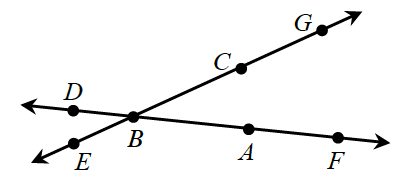### Home > GC > Chapter 5 > Lesson 5.3.3 > Problem5-94

5-94.Examine the diagram at right. Which angle below is another name for $∠ABC$? Note: More than one solution is possible.

1. $∠ABE$

1. $∠GBD$

1. $∠FBG$

1. $∠EBC$

1. none of these

Trace the points in order to see if they make the same angle as ∠ABC.

C